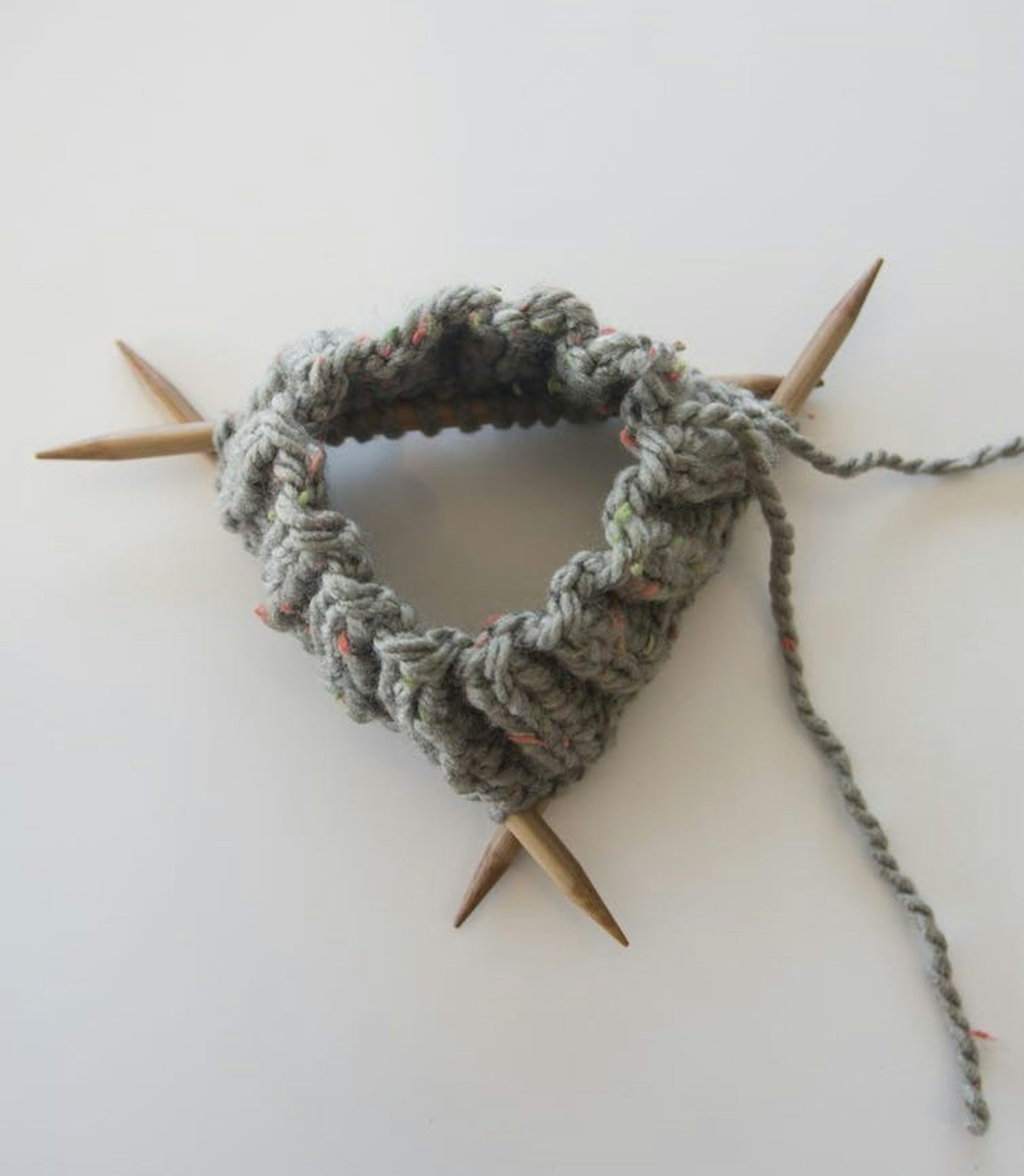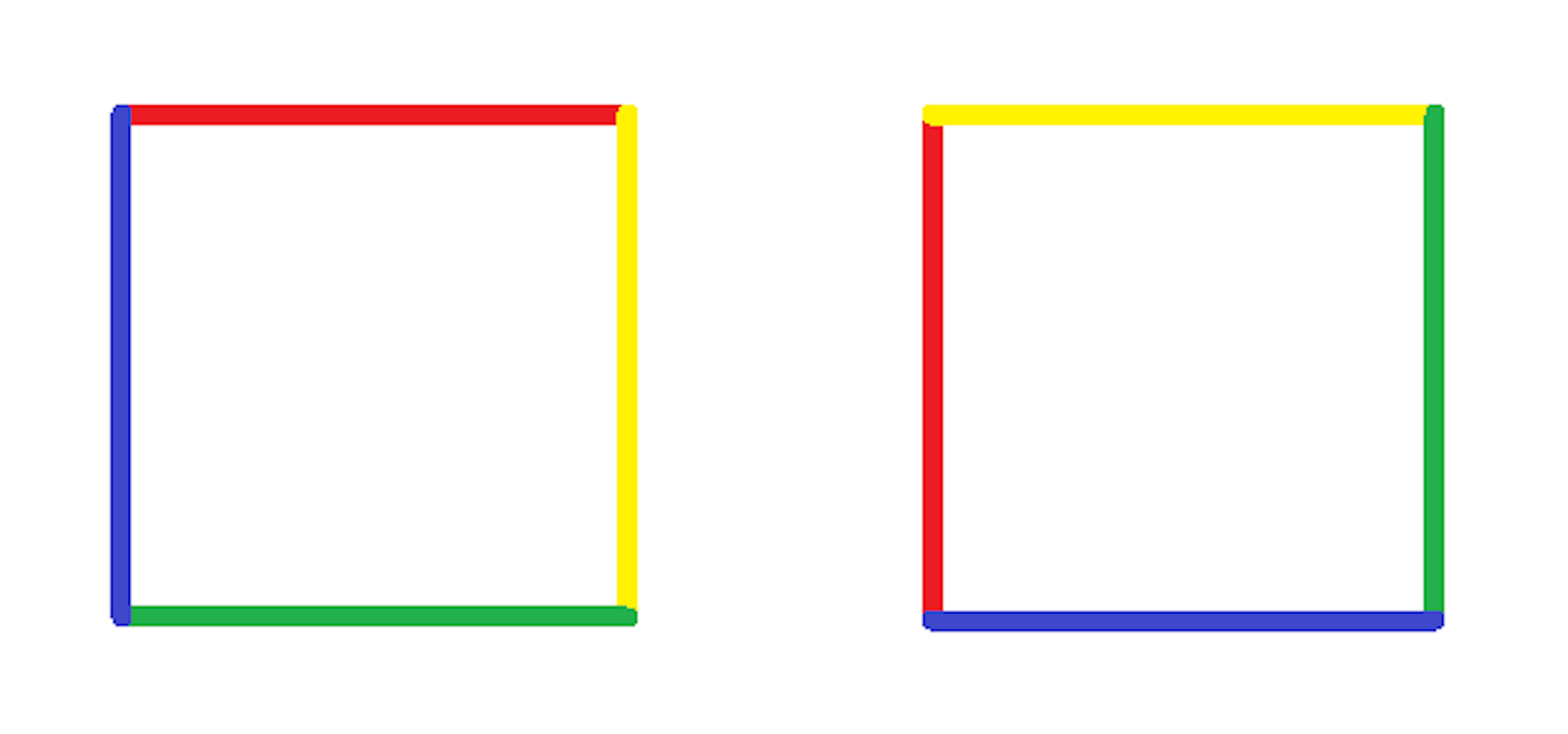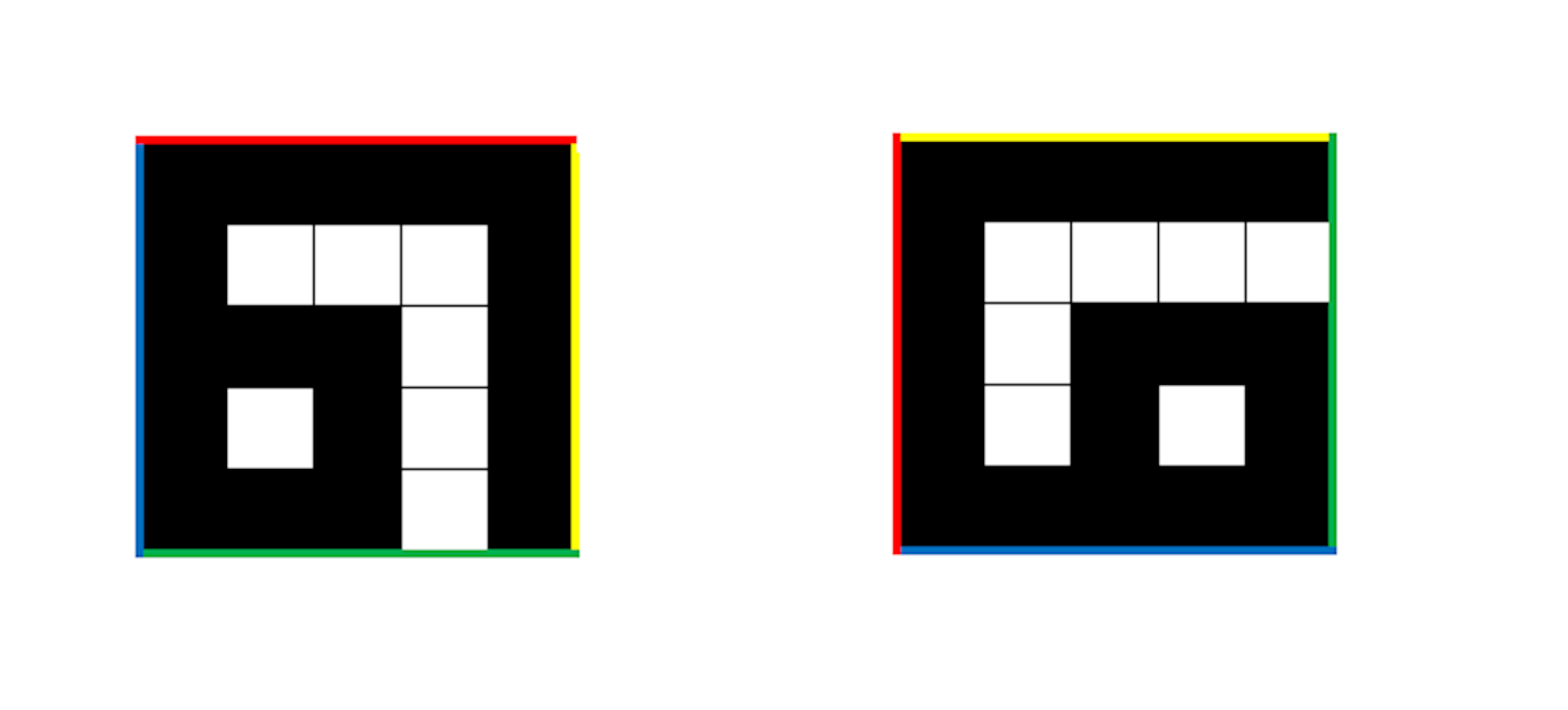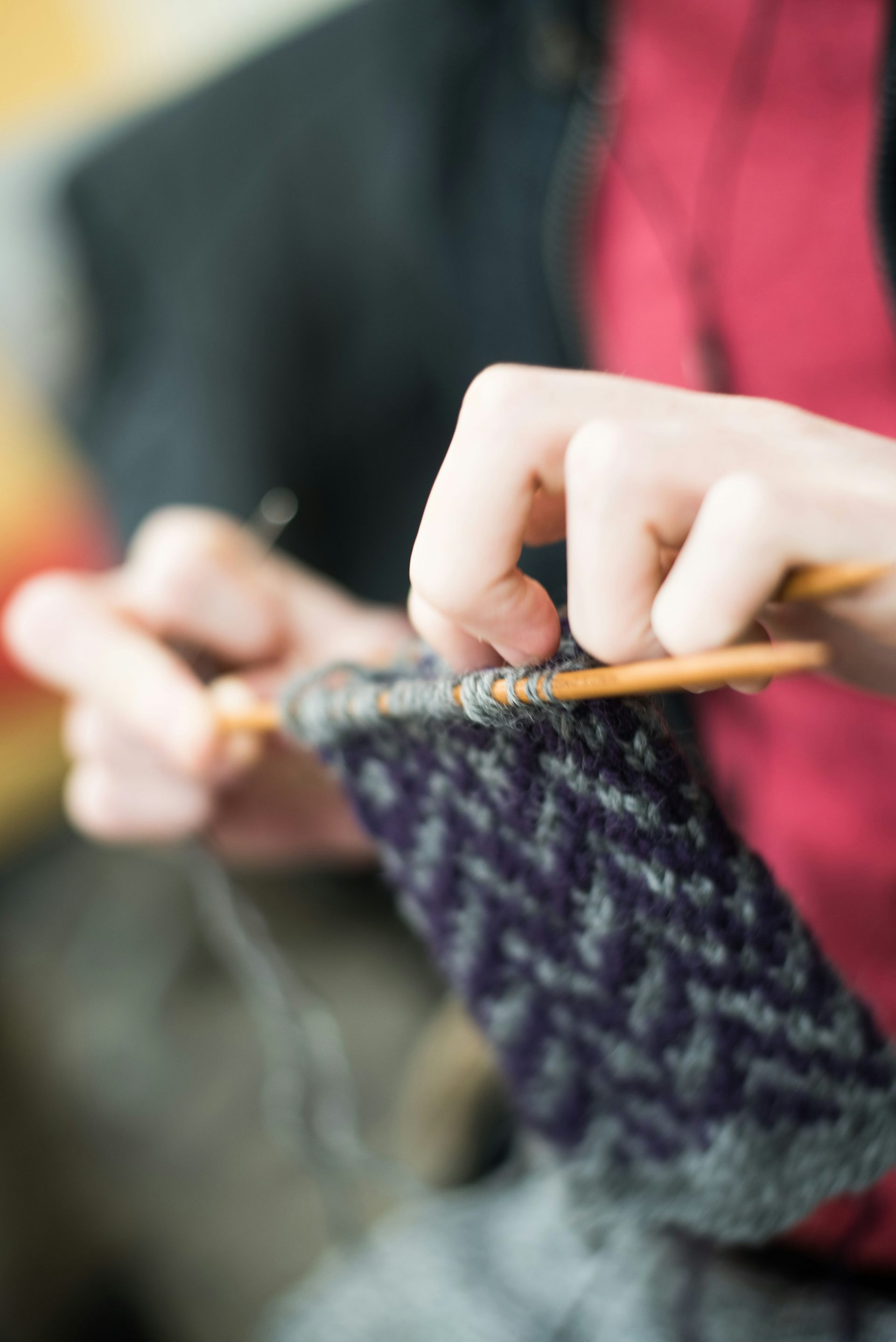Why I teach math through knittingMath in yarn. Carthage College, CC BY-SA

One snowy January day, I asked a classroom of college students to tell me the first word that came to mind when they thought about mathematics. The top two words were “calculation” and “equation.”

When I asked a room of professional mathematicians the same question, neither of those words were mentioned; instead, they offered phrases like “critical thinking” and “problem-solving.”

This is unfortunately common. What professional mathematicians think of as mathematics is entirely different from what the general population thinks of as mathematics. When so many describe mathematics as synonymous with calculation, it’s no wonder we hear “I hate math” so often.

So I set out to solve this problem in a somewhat unconventional way. I decided to offer a class called “The Mathematics of Knitting” at my institution, Carthage College. In it, I chose to eliminate pencil, paper, calculator (gasp) and textbook from the classroom completely. Instead, we talked, used our hands, drew pictures and played with everything from beach balls to measuring tapes. For homework, we reflected by blogging. And of course, we knit.

Same but different

One crux of mathematical content is the equation, and crucial to this is the equal sign. An equation like x = 5 tells us that the dreaded x, which represents some quantity, has the same value as 5. The number 5 and the value of x must be exactly the same.

A typical equal sign is very strict. Any small deviation from “exactly” means that two things are not equal. However, there are many times in life where two quantities are not exactly the same, but are essentially the same by some meaningful criteria.

Imagine, for example, that you have two square pillows. The first is red on top, yellow on the right, green on bottom and blue on the left. The second is yellow on the top, green on the right, blue on bottom, and red on the left.

The pillows aren’t exactly the same. One has a red top, while one has a yellow top. But they’re certainly similar. In fact, they would be exactly the same if you turned the pillow with the red top once counterclockwise.Rotating two square pillows. Sara Jensen

How many different ways could I put the same pillow down on a bed, but make it look like a different one? A little homework shows there are 24 possible colored throw pillow configurations, though only eight of them can be obtained from moving a given pillow.

Students demonstrated this by knitting throw pillows, consisting of two colors, from knitting charts.A knitting chart for a throw pillow. Sara Jensen

The students created square knitting charts where all eight motions of the chart resulted in a different-looking picture. These were then knit into a throw pillow where the equivalence of the pictures could be demonstrated by actually moving the pillow.

Rubber sheet geometry

Another topic we covered is a subject sometimes referred to as “rubber sheet geometry.” The idea is to imagine the whole world is made of rubber, then reimagine what shapes would look like.

Let’s try to understand the concept with knitting. One way of knitting objects that are round – like hats or gloves – is with special knitting needles called double pointed needles. While being made, the hat is shaped by three needles, making it look triangular. Then, once it comes off the needles, the stretchy yarn relaxes into a circle, making a much more typical hat.Knitting to learn. Carthage College, CC BY-SA

This is the concept that “rubber sheet geometry” is trying to capture. Somehow, a triangle and a circle can be the same if they’re made out of a flexible material. In fact, all polygons become circles in this field of study.

If all polygons are circles, then what shapes are left? There are a few traits that are distinguishable even when objects are flexible – for example, if a shape has edges or no edges, holes or no holes, twists or no twists.

One example from knitting of something that is not equivalent to a circle is an infinity scarf. If you want to make a paper infinity scarf at home, take a long strip of paper and glue the short edges together by attaching the top left corner to the bottom right corner, and the bottom left corner to the top right corner. Then draw arrows pointing up the whole way around the object. Something cool should happen.

Students in the course spent some time knitting objects, like infinity scarves and headbands, that were different even when made out of flexible material. Adding markings like arrows helped visualize exactly how the objects were different.

Different flavorsIf more mathematicians were able to set aside classical techniques, it seems possible the world could overcome the prevailing misconception that computation is the same as mathematics. And just maybe, a few more people out there could embrace mathematical thought; if not figuratively, then literally, with a throw pillow.